﻿ Solution of the Burgers Equation by the Method of Lines

### Solution of the Burgers Equation by the Method of Lines

J. Biazar, Z. Ayati, S. ShahbaziOPEN ACCESSPEER-REVIEWED

## Solution of the Burgers Equation by the Method of Lines

J. Biazar1, Z. Ayati2,, S. Shahbazi1

1Department of Applied Mathematics, Faculty of Mathematical Sciences, University of Guilan, Rasht, Iran

2Department of Engineering sciences, Faculty of Technology and Engineering East of Guilan, University of Guilan, Rudsar-Vajargah, Iran

### Abstract

The method of lines (MOL), as a semi analytical procedure, is well known to experts in computational techniques in electromagnetic. The range of applications of the method has increased dramatically in the past few years. Nevertheless, there is no introductory paper to initiate to the method a beginner. This paper has been illustrated the application of the MOL to solve burgers equation. Three numerical examples are presented to illustrate the procedure. The obtained results have been compared with analytical solutions and are satisfactory.

• Biazar, J., Z. Ayati, and S. Shahbazi. "Solution of the Burgers Equation by the Method of Lines." American Journal of Numerical Analysis 2.1 (2014): 1-3.
• Biazar, J. , Ayati, Z. , & Shahbazi, S. (2014). Solution of the Burgers Equation by the Method of Lines. American Journal of Numerical Analysis, 2(1), 1-3.
• Biazar, J., Z. Ayati, and S. Shahbazi. "Solution of the Burgers Equation by the Method of Lines." American Journal of Numerical Analysis 2, no. 1 (2014): 1-3.

 Import into BibTeX Import into EndNote Import into RefMan Import into RefWorks

### 1. Introduction

The basic idea of the method of line is to replace the spatial (boundary value) derivatives in the PDE with algebraic approximations [1, 2, 3]. Once this is done, the spatial derivatives are no longer stated explicitly in terms of the spatial independent variables. Thus, in effect only the initial value variable, typically time in a physical problem, remains. In other words, with only one remaining independent variable, we have a system of ODEs that approximate the original PDE. The challenge, then, is to formulate the approximating system of ODEs. Once this is done, we can apply any integration algorithm for initial value ODEs to compute an approximate numerical solution to the PDE. Thus, one of the salient features of the MOL is the use of existing, and generally well established, numerical methods for ODEs.

### 2. Burger's Equation

Consider Burgers' equation in the form(1)

Where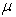is positive parameter and homogeneous boundary conditions are used. A study of the properties of Burger's equation is of great importance due to the equation's applications in the approximate theory of flow through a shock wave traveling in a viscous fluid and the Burger's model turbulence. Burger's equation is a very good example for several reasons It is nonlinear.

Ÿ The exact solution of the PDE is known.

Ÿ  It can be thought of as hyperbolic problem with artificial diffusion for small.

Ÿ  It is sometimes used in boundary layer calculation for the flow of viscous fluid.

Ÿ  It is very nearly a standard test problem for PDE solvers.

This equation is solved recently by different methods such as in [4, 5, 6, 7]. Apply the method of lines to equation (2.1) we discretized the partial derivatives with respect to the space variables to result in approximating system of ODEs in the variable t and in the MOL approach, the ODEs are integrated directly with a standard code for the task. The simplest method of spatial discretization is to discretize along the x axis with a uniform mesh and to replace all spatial derivatives in (2.1) by finite difference.

Letand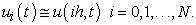the resulting is a system of ODEs, for the method of lines approach to solving (2.1).

### 3. Method of Lines

On a uniform step size mesh with step size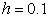second-order centered differences for the first and second derivatives of a function are commonly given by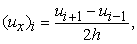(2)(3)

By substituting (2) and (3) into (1) and takingwe have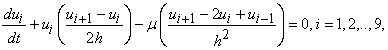(4)

Or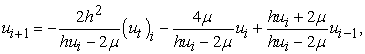(5)

Matrix form of equation (5) can be written as followsSystem (5) for each i, is ordinary differential equation and it consists of 9 equation and 9 unknowns.

### 4. Numerical Results

Example 1. Consider the Eq.(1) with following initial and boundary conditions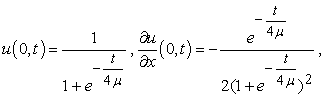whereWith the exact solutions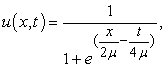(6)

Where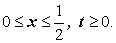Let’sBy taking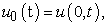we getThe values of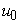andin various time can be obtained as followsTable 1 shows the numerical solving Eq.(1) by MOL and absolute errors whenfrom 0.0 to 0.5 and t is 0, 0.25 and 0.5.

#### Table 1. The solution of u(x,t) for different values of x and t

Example2. Let us consider the Eq. (2-1) with following initial and boundary conditionsThe exact solution of the equation as followsWhere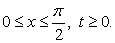We haveAs in the previous example, the value of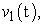can be obtained as follows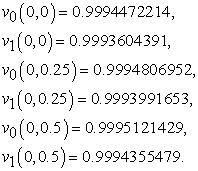The results of numerical solving Eq.(1) by MOL and errors at each point are presented in Table 2.

### 5. Conclusion

In this paper, burgers equation has been solved by the method of lines and the desired numerical solution has been achieved. The obtained numerical solutions have been compared with the exact solution, and the results show the efficiency of the method. In the solved examples, the solutions of the burgers equation are recorded for### References

  J. Biazar and N. nomidi, “solution of the Emden-Fowler equation by the method of lines”, Journal of nature science and sustainable, 7(2) (2013) 45-55.In article  W. E, Schiesser, “The Numerical Methods of Lines – Integration of Partial Differential Equations”, Acadimic Press, San Diego, 1991.In article  R. Knapp., “A Method of Lines Framework in Mathematica”, JNAIAM, 3(1) (2008) 43-59.In article  Byrne, G.D. and A.C. Hindmarsh, “Stif ODE Solvers: A review of Current and Coming Attractions”, Journal of Computational physics, 70 (1987) 1-62.In article CrossRef  C. Mamaloukas, S. Spartalis, “Decomposition method in Comparison with Numerical Solutions of Burger's equation”, Ratio Mathematica, 18 (2008) 51-61.In article  C. Mamaloukas, K. Haldar, and H.P. Mazumdar, “Application of double decomposition to pulsatile flow”, Journal of Computational &Applied Mathematics, 10(2) (2002) 193-207.In article CrossRef  M. Javidi, “A Numerical Solution of Burger's equation based on modified extended BDF scheme”, Int. Math.Forum, 1(32) (2006) 1565-1570.In article